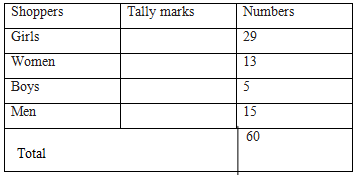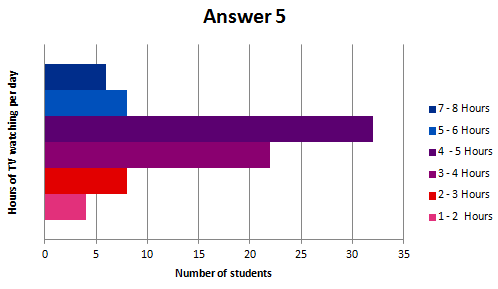Study Materials: ncert solutions

Our ncert solutions for Exercise 5.1 Class 8 maths 5. Data Handling - ncert solutions - Toppers Study is the best material for English Medium students cbse board and other state boards students.

Solutions ⇒ Class 8th ⇒ Mathematics ⇒ 5. Data Handling

# Exercise 5.1 Class 8 maths 5. Data Handling - ncert solutions - Toppers Study

Topper Study classes prepares ncert solutions on practical base problems and comes out with the best result that helps the students and teachers as well as tutors and so many ecademic coaching classes that they need in practical life. Our ncert solutions for Exercise 5.1 Class 8 maths 5. Data Handling - ncert solutions - Toppers Study is the best material for English Medium students cbse board and other state boards students.

## Exercise 5.1 Class 8 maths 5. Data Handling - ncert solutions - Toppers Study

CBSE board students who preparing for class 8 ncert solutions maths and Mathematics solved exercise chapter 5. Data Handling available and this helps in upcoming exams 2023-2024.

### You can Find Mathematics solution Class 8 Chapter 5. Data Handling

• All Chapter review quick revision notes for chapter 5. Data Handling Class 8
• NCERT Solutions And Textual questions Answers Class 8 Mathematics
• Extra NCERT Book questions Answers Class 8 Mathematics
• Importatnt key points with additional Assignment and questions bank solved.

NCERT Solutions do not only help you to cover your syllabus but also will give to textual support in exams 2023-2024 to complete exercise 5.1 maths class 8 chapter 5 in english medium. So revise and practice these all cbse study materials like class 8 maths chapter 5.1 in english ncert book. Also ensure to repractice all syllabus within time or before board exams for ncert class 8 maths ex 5.1 in english.

See all solutions for class 8 maths chapter 5 exercise 5 in english medium solved questions with answers.

### Exercise 5.1 class 8 Mathematics Chapter 5. Data Handling

• Exercise 5.1 Class 8 Maths 5. Data Handling - Ncert Solutions - Toppers Study
• Class 8 Ncert Solutions
• Solution Chapter 5. Data Handling Class 8
• Solutions Class 8
• Chapter 5. Data Handling Exercise 5.1 Class 8

## Exercise 5.1 Class 8 maths 5. Data Handling - ncert solutions - Toppers Study

1.   For which of these would you use a histogram to show the data? (a)   The number of letters for different areas in a postman’s bag.

(b)   The height of competitors in an athletics meet.

(c)   The number of cassettes produced by 5 companies.

(d)   The number of passengers boarding trains from 7:00 a.m. to 7:00 p.m. at a station.

Give reasons for each.

Ans.

In the case of the data given in alternative (b) and (d), we will use histogram as we can divide

The given data in class intervals. In case of alternative (a) and (c), we do not know about the numbers of letter of different areas and the number of cassettes produces by given companies. We don’t have any approximate idea about it. Therefore, we cannot define class intervals for this data and thus we will note use a histogram.

2.   The shoppers who come to a departmental store are marked as: man (M), woman (W), boy (B) or girl (G). The following list gives the shoppers who came during the first hour in the morning:

W W W G B W W M G G M M W

W W W G B M W B G G M W W M M W W W M W B W G M W W W G W M M W W M W G W M G W M M B G G W

Ans:

By observing the data above, we can make a frequency distribution table as follows.3.   The weekly wages (in `) of 30 workers in a factory are.

830, 835, 890, 810, 835, 836, 869, 845, 898, 890, 820, 860, 832, 833, 855, 845,

804, 808, 812, 840, 885, 835, 835, 836, 878, 840, 868, 890, 806, 840

Using tally marks make a frequency table with intervals as 800–810, 810–820 and so on.

Ans:1. 830-840 is the group which has maximum number of workers.
2. The worker who earn more than Rs 850 are the number of workers who fall in the group of 800-810 or 810-820 or 820-830 or 830-840 or 840-850 Hence the total number of workers earning less than 850 will be the sum of the numbers of all these workers i.e. 3 + 2 + 1 + 9 + 5 = 20

5.   The number of hours for which students of a particular class watched television during holidays is shown through the given graph.

1. for how many hours did the maximum number of students watch TV?
2.   How many students watched TV for less than 4 hours?
3. How many students spend more than 5 hours in watching TV?

1. From the graph, it can be observe that the maximum number of students watched TV for 4 – 5 hours.
2. The students who watched TV for less than 4 hours are students who watched TV for  1-2 hours or 2-3 hours or 3-4 hours.

Hence the total number of students = 4 + 8 + 22 = 34

1. The students who watched TV for more than 5 hours are students who watched TV for 5-6 hours or 6-7 hours.

Hence, total number of students = 8 + 6 =  14##### Other Pages of this Chapter: 5. Data Handling

Important Study materials for classes 06, 07, 08,09,10, 11 and 12. Like CBSE Notes, Notes for Science, Notes for maths, Notes for Social Science, Notes for Accountancy, Notes for Economics, Notes for political Science, Noes for History, Notes For Bussiness Study, Physical Educations, Sample Papers, Test Papers, Mock Test Papers, Support Materials and Books.Mathematics Class - 11th

NCERT Maths book for CBSE Students.

books

## Study Materials List:

##### Solutions ⇒ Class 8th ⇒ Mathematics
1. Rational Numbers
2. Linear Equations in One Variable
4. Practical Geometry
5. Data Handling
6. Squares and Square Roots
7. Cubes and Cube Roots
8. Comparing Quantities
9. Algebraic Expressions and Identities
10. Visualising Solid Shapes
11. Mensuration
12. Exponents and Powers
13. Direct and Inverse Proportions
14. Factorisation
15. Introduction to Graphs
16. Playing with Numbers

New Books